# AP Physics B : Understanding Period and Amplitude

## Example Questions

### Example Question #2 : Ap Physics 2

Two in-phase waves travelling in the same direction with the same period have amplitudes of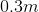and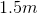, respectively. A third, out-of-phase wave with the same period as the other two has an amplitude of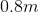. What will be the amplitude of the final resultant wave when these three are aligned?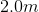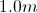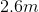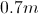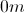Explanation:

The amplitudes of in-phase waves add in what is called constructive interference. We can find the resultant amplitude of the first two waves by adding their individual amplitudes.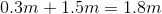The third wave is out of phase, meaning that it will contribute destructive interference when the three waves align. We subtract the amplitude of the third wave from the total of the first two.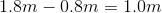This is the final amplitude of the resultant wave.